Classifying triangles

Chapter 5 Class 6 Understanding Elementary Shapes
Concept wise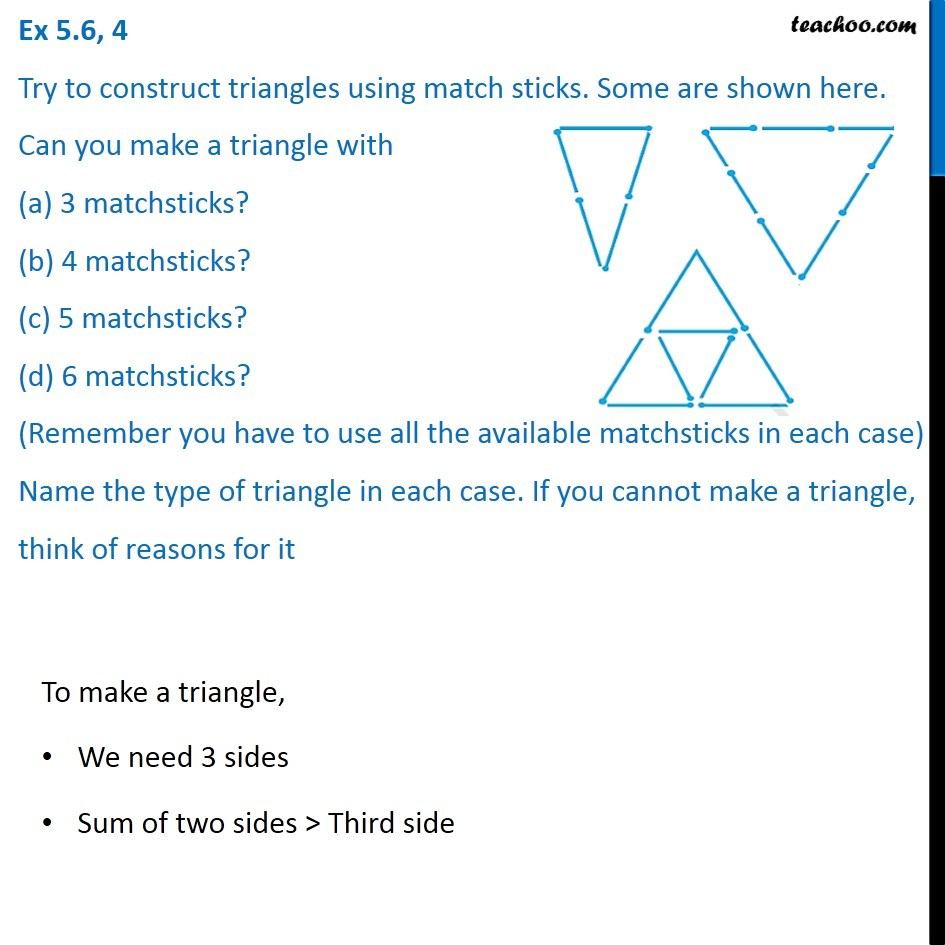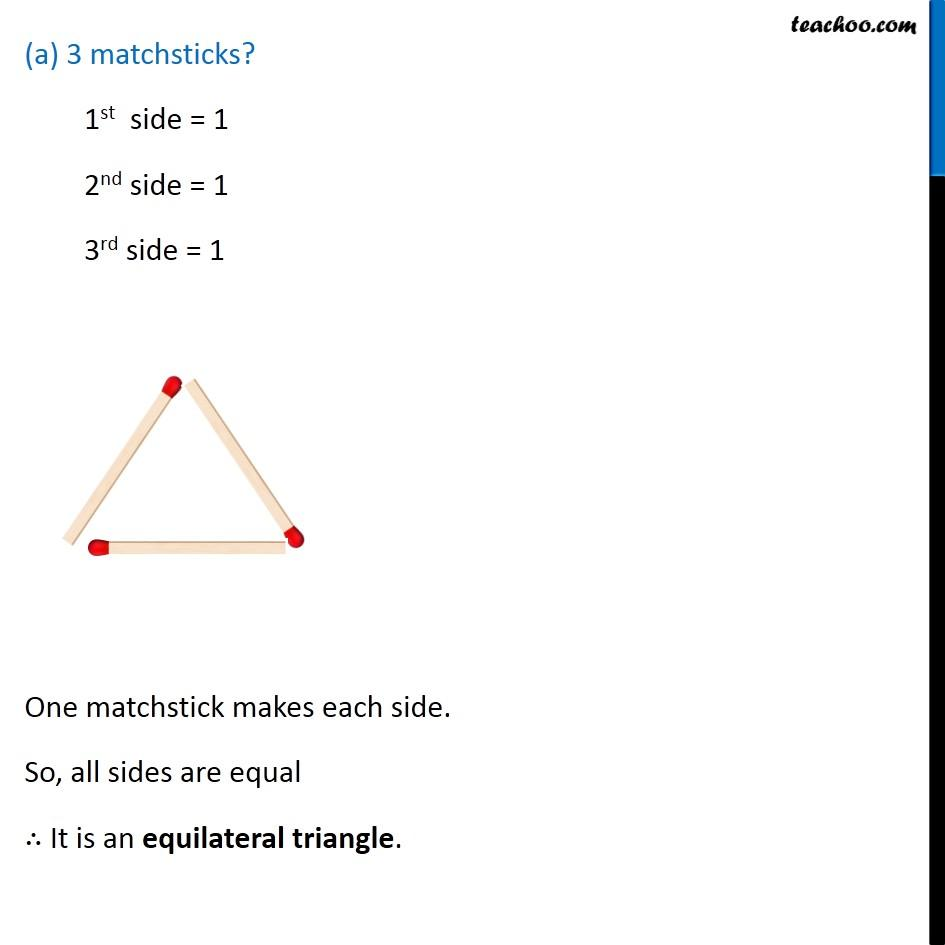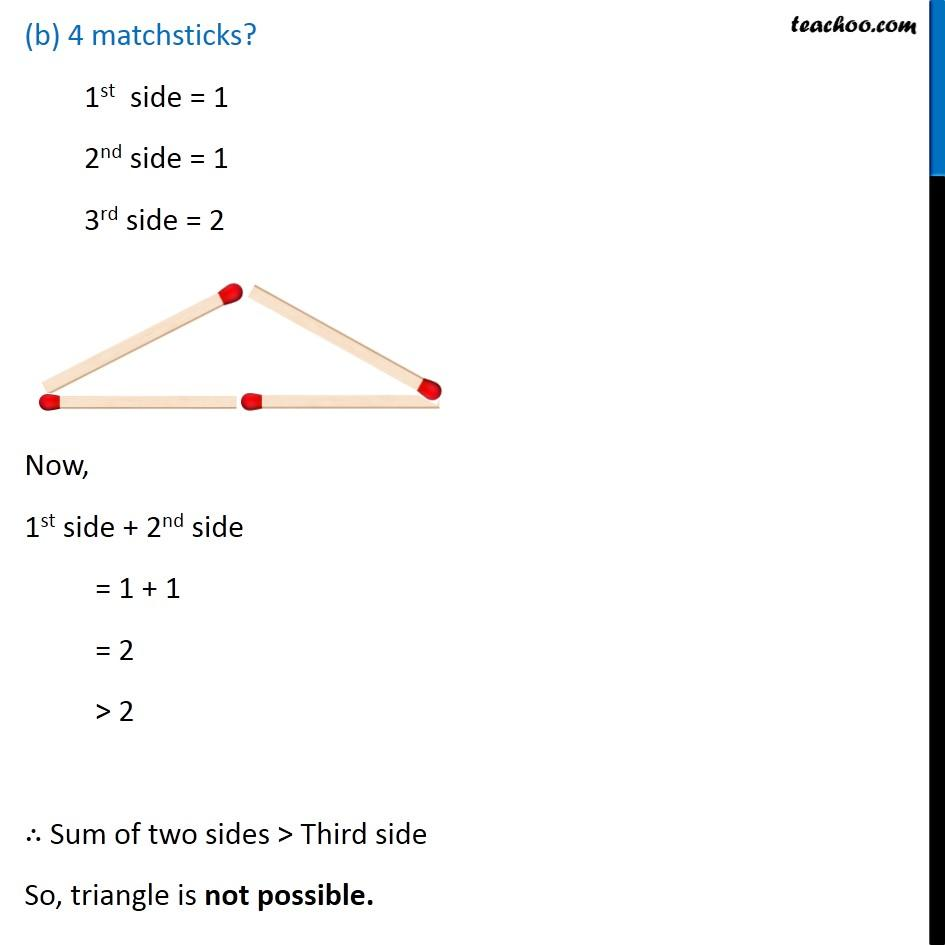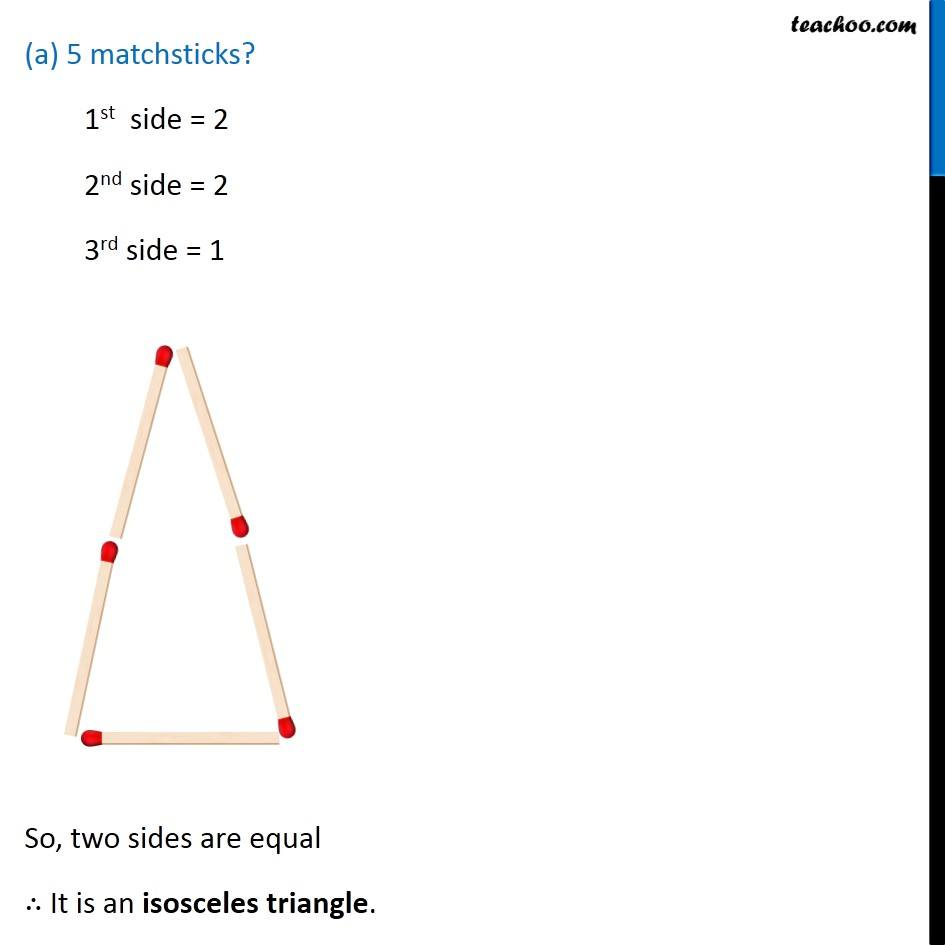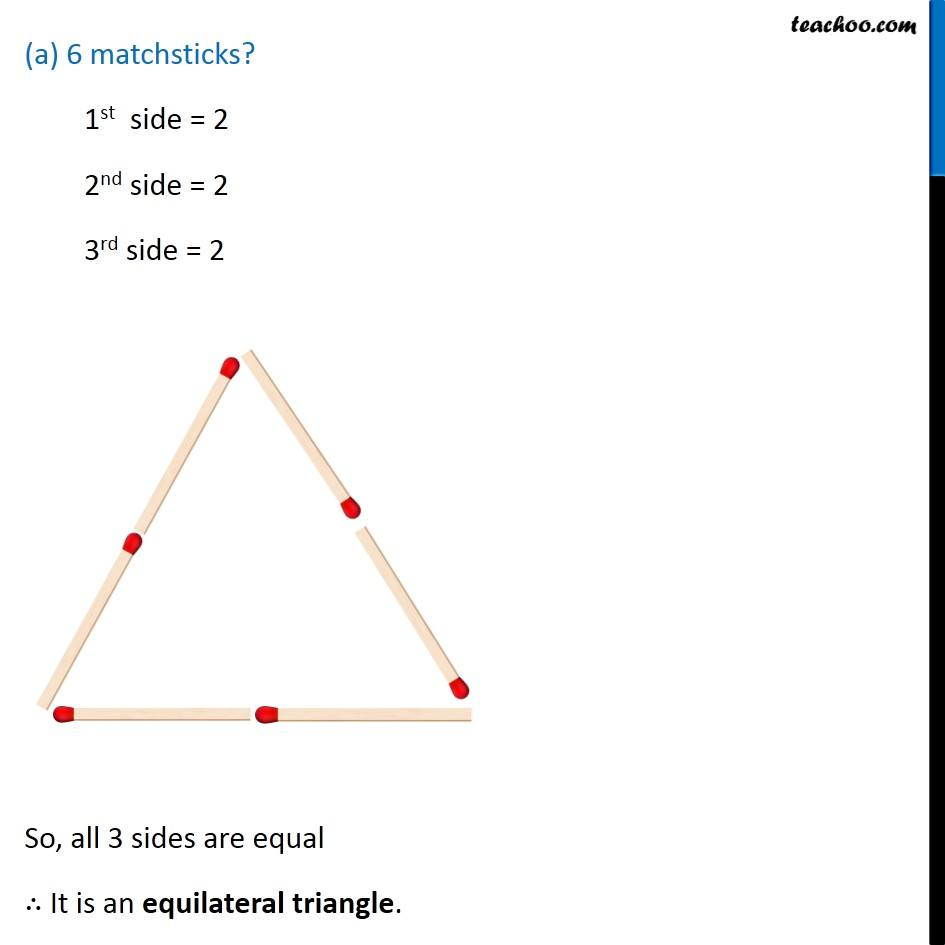Learn in your speed, with individual attention - Teachoo Maths 1-on-1 Class

### Transcript

Ex 5.6, 4 Try to construct triangles using match sticks. Some are shown here. Can you make a triangle with (a) 3 matchsticks? (b) 4 matchsticks? (c) 5 matchsticks? (d) 6 matchsticks? (Remember you have to use all the available matchsticks in each case) Name the type of triangle in each case. If you cannot make a triangle, think of reasons for it To make a triangle, We need 3 sides Sum of two sides > Third side (a) 3 matchsticks? 1st side = 1 2nd side = 1 3rd side = 1 One matchstick makes each side. So, all sides are equal ∴ It is an equilateral triangle. (b) 4 matchsticks? 1st side = 1 2nd side = 1 3rd side = 2 Now, 1st side + 2nd side = 1 + 1 = 2 > 2 ∴ Sum of two sides > Third side So, triangle is not possible. (a) 5 matchsticks? 1st side = 2 2nd side = 2 3rd side = 1 So, two sides are equal ∴ It is an isosceles triangle. (a) 6 matchsticks? 1st side = 2 2nd side = 2 3rd side = 2 So, all 3 sides are equal ∴ It is an equilateral triangle.